Actions

An Abelian group associated with an associative ring in the following manner. It was introduced by J.H.C. Whitehead . Letbe an associative ring with unit element and let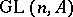be the group of invertible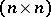-matrices over. There are natural imbeddings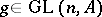goes to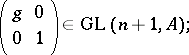let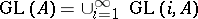. A matrix differing from the identity matrix in a single non-diagonal entry is called an elementary matrix. The subgroup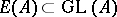generated by all elementary matrices coincides with the commutator group of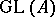. The commutator quotient group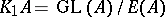is called the Whitehead group of the ring. Letbe the element corresponding to the matrixIt has order 2. The quotient group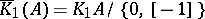is called the reduced Whitehead group of the ring.

Let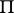be a multiplicative group and letbe its group ring over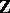. There is a natural homomorphism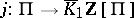coming from the inclusion of. The quotient groupis called the Whitehead group of the group.

Given a homomorphism of groups, there is a natural induced homomorphism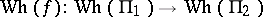, such thatfor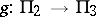. Thus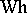is a covariant functor from the category of groups into the category of Abelian groups. If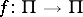is an inner automorphism, then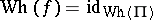.

The Whitehead group of the fundamental group of a space is independent of the choice of a base point and is essential for the definition of an important invariant of mappings, the Whitehead torsion.